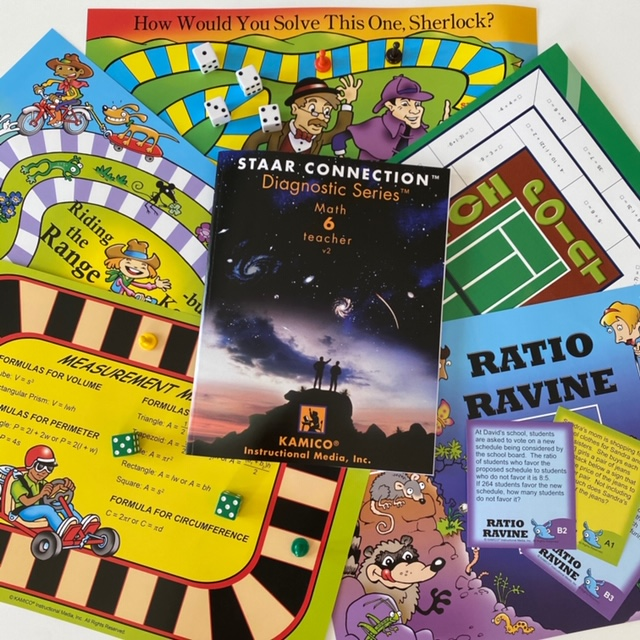#### QUICK START PACK - Grade 6 Math

\$110.00 Qty: (0 in cart)

SKU: QSP6M

Includes the STAAR CONNECTION™ Diagnostic Series™ Grade 6 Math teacher edition; Game Gallery® (9 - 1 of each of the grade 6 TEKS-based math board games) 15% discount

### Details

Quick Start Pack

Math

STAAR CONNECTIONDiagnostic Series

 Item # Description ISBN Qty STDI6MV2T Gr 6 Math v2 - Teacher 978-1-62426-240-1 1

Game Gallery® Gr 6 Math Games

 Item # Game Title and TEKS Qty GG6MMES 400-Meter Expression Sprint/Relay 6(7)(A) Expressions, equations, and relationships. Evaluate expressions and generate equivalent numerical expressions. 1 GG6MCYM Coordinate Your Moves 6(11) Measurement and data. Graph points in all four quadrants using ordered pairs of rational numbers. 1 GG6MF Fivers 6(4)(C) Proportionality. Develop an understanding of proportional relationships in problem situations. 1 GG6MHWY How Would You Solve This One, Sherlock? 6(6)(C) Expressions, equations, and relationships. Represent a given situation using descriptions, tables, graphs, and equations. 1 GG6MMP Match Point 6(3)(C) Number and operations. Represent integer operations with concrete models and connect the actions to the models to standardized algorithms. 1 GG6MMM2 Measure Match 6(4)(H) Proportionality. Convert units within a measurement system. 1 GG6MMM Measurement Mile 6(8)(D) Expressions, equations, and relationships. Determine solutions for problems involving area of rectangles, parallelograms, trapezoids, and triangles and volume of rectangular prisms. 1 GG6MRR Ratio Ravine 6(3)(E) Number and operations. Multiply and divide positive rational numbers - ratios and rates. 1 GG6MRTR Riding the Range - but Keep off the Median! 6(12)(B,C,D) Measurement and data. Use the graphical representation of numeric data to describe the center, spread, and shape of the data distribution. Summarize numeric and categorical data with numerical and graphical summaries. 1